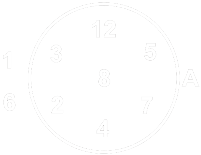Home > Math Shortcuts > Statistics Math Formulas

# Statistics Math Formulas

## Statistics Math Formulas

Statistics Math Formulas :
SETS :A = {2, 3, 4, 7, 8, 9, 12}

3 ∈ A

5 ∉ A

SUBSET:B = {3, 8, 9} ⇒ B ⊆ A

C = {1, 5} ⇒ C ⊄ A

STATISTICS :

MEAN : The mean value is obtained the arithmetic mean or average of a set of numbers is expected value.
The mean value is calculated by adding up all the values, and then dividing that sum by the number of values .Mean = Sum of all data values / Number of data values

Symbolically ,

Where  (read as ” x bar”) is the mean of the set of x values, Σ x is the sum of all the x values, and n is the number of x values.

MEDIAN : The median is the middle value in a set of values. So to find the midian you need to order the numbers from largest to smallest and then you have to choose the value in the middle.

MODE : Mode is the value that the highest frequency in the data set.means values that occur most frequently and there can be more than one mode in a set.

numerical value that occurs most of the times.
F (Xmode) = max

INTERSECTION :In intersection A ∩ B of two sets A and B is the set that contains all elements of B also belong to A (or similarly all elements of B that also belong to A) but no other elements. The symbol intersection is inverted U.

If Set A contain element A = {1,2,3} and set B contains B = {2,3,4} and the element in having common ares 2 and 3 and this intersection area formed a new set containing 2 and 3.

UNION :The union of two sets A and B includes all elements which are members of either A or B. If sets A and B have any elements in common then this elements which are members of both sets are only include one in the union.

For example : If sets A contains the elements 1,2, and 3 and set B contains 2,3 and 4 the elements which are members of A or B are 1,2,3 and 4. This form a new set containing 1,2,3 and 4. when we write the union 2 and 3 are only listed once.

RELATIVE COMPLEMENT OF A IN B :The relative complement of A in B denoted, B \ A, is the set of elements in B but not in A.

Symbolically :B \ A = {x |  x ∈ B ∧ x ∉ A}

ABSOLUTE COMPLEMENT :In a Set theory a complement of a set A refers to things not in A.

SYMMETRIC DIFFERENCE :Operations on sets :

A ∪ A = A

A ∩ A = A

A ∪ B = B ∩  A

A ∩ B = B ∩ A

(A ∪ B) ∪ C = A ∪ (B ∪ C)

(A ∩ B) ∩ C = A ∩ (B ∩ C)

∪′ = ø

(A′)′ = A

A ∩ ø = ø

A ∩ U = A

A ∩ A′ = ø

(A ∪ B)′ = A′ ∩ B′

(A ∩ B)′ = A′ ∪ B′

1.